Ask your WordPress questions! Pay money and get answers fast!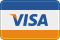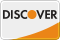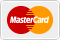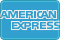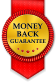# Total hours within range WordPress

• SOLVED

I have two date in the following format,

``` F jS, Y H:i:s [after] => May 11th, 2018 00:00:00 [before] => May 11th, 2018 08:00:00 ```

and I need to find out how many hours of above are within '06:00:00 to 23:00:00' this time span wont change. However sometimes the after or before will span more than one day :eg

``` [after] => May 11th, 2018 23:00:00 [before] => May 12th, 2018 08:00:00 ```

I think a function I can pass the dates to would be best in my case.

2018-05-16

This is crude but might be sufficient:

``` \$a = 'May 11th, 2018 23:00:00'; \$b = 'May 12th, 2018 08:00:00'; echo 'Hours='. get_hours( \$a, \$b ); function get_hours( \$date1, \$date2 ) { return abs( strtotime(\$date1) - strtotime(\$date2) ) / HOUR_IN_SECONDS; } ```

I may not have been clear john sorry, I need to know how many of the hours between \$a and \$b

\$a = 'May 11th, 2018 23:00:00';
\$b = 'May 12th, 2018 08:00:00';

fall within "'06:00:00 to 23:00:00'"

\$a = 'May 11th, 2018 23:00:00';
\$b = 'May 12th, 2018 08:00:00';

fall within "'06:00:00 to 23:00:00'"

so in this case the amount would be 2 hours.

2018-05-16

2018-05-17

``` function timd_get_hours(\$start, \$end) { \$output = round( abs( strtotime(\$end) - strtotime(\$start) ) / 3600 ); return \$output; } //sample \$a = "May 11th, 2018 00:00:00"; \$b = "May 11th, 2018 23:00:00"; \$c = "May 12th, 2018 08:00:00"; echo( timd_get_hours(\$a, \$b) ); // output = 23 echo( timd_get_hours(\$b, \$c) ); // output = 9 ```

2018-05-17

Hello, may I know your php version?

I have this function, but will only work on php greater than 5.6.5.

http://sandbox.onlinephpfunctions.com/code/fce8a84b651811b0898d1e2ee24d499904aa5cd1

``` \$startDate1 = 'May 11th, 2018 00:00:00'; \$endDate1 ='May 11th, 2018 08:00:00'; \$startTime = '06:00:00'; \$endTime = '23:00:00'; echo dateOverlapsInHours( \$startDate1, \$endDate1, \$startTime, \$endTime ); // result 2 \$startDate1 = 'May 11th, 2018 00:00:00'; \$endDate1 ='May 12th, 2018 08:00:00'; \$startTime = '06:00:00'; \$endTime = '23:00:00'; echo dateOverlapsInHours( \$startDate1, \$endDate1, \$startTime, \$endTime ); // result 19 \$startDate1 = 'May 1st, 2018 00:00:00'; \$endDate1 ='May 1st, 2018 08:00:00'; \$startTime = '06:00:00'; \$endTime = '23:00:00'; echo dateOverlapsInHours( \$startDate1, \$endDate1, \$startTime, \$endTime ); // result 2```

2018-05-17

Try this please, tested and working:

In functions.php:
```function get_the_hours(\$one, \$two) { \$time1 = new DateTime(date("H:i:s",strtotime(\$one))); //Date one time \$time2 = new DateTime(date("H:i:s",strtotime(\$two))); //Date two time \$date1 = new DateTime(date("Y-m-d",strtotime(\$one))); //Date one \$date2 = new DateTime(date("Y-m-d",strtotime(\$two))); //Date two \$start = new DateTime("06:00:00"); // hour range start \$end = new DateTime("23:00:00"); // hour range end \$hours_range = \$end->diff(\$start)->format("%h"); // hours range \$full_day_hours = (\$date2->diff(\$date1)->format("%a")-1)*\$hours_range; \$first_day_hours = min(\$hours_range,max(0,\$end->diff(\$time1)->format("%h"))); \$last_day_hours = min(\$hours_range,max(0,\$time2->diff(\$start)->format("%h"))); \$hours = \$first_day_hours + \$full_day_hours + \$last_day_hours; echo \$hours; }```

```\$first = 'May 11th, 2018 23:00:00'; \$last = 'May 12th, 2018 08:00:00'; get_the_hours(\$first, \$last);```

Hi, did you check this answer?

2018-05-16

Hi!

Try this code:

```function get_hours( \$date1, \$date2 ) { \$day_start_time = '06:00:00'; \$day_end_time = '23:00:00'; \$whole_day_hours = ( strtotime( \$day_end_time ) - strtotime( \$day_start_time ) ) / 3600; // hours between 06:00:00 to 23:00:00 \$t1 = strtotime( \$date1 ); \$t2 = strtotime( \$date2 ); \$start_date = date( 'Y-m-d', \$t1 ); \$end_date = date( 'Y-m-d', \$t2 ); \$d1 = new DateTime( \$start_date ); \$d2 = new DateTime( \$end_date ); \$days_diff = \$d1->diff( \$d2 )->days; if ( 0 === \$days_diff ) { \$result_diff = \$whole_day_hours + min( 0, ( ( strtotime( \$start_date . \$day_start_time ) - \$t1 ) / 3600 )) + min(0, ( \$t2 - strtotime( \$end_date . \$day_end_time ) ) / 3600 ); } else { \$first_day_hours = \$whole_day_hours + min( 0, ( strtotime( \$start_date . \$day_start_time ) - \$t1 ) / 3600 ); \$last_day_hours = max( 0, \$whole_day_hours + ( \$t2 - strtotime( \$end_date . \$day_end_time ) ) / 3600 ); \$result_diff = \$first_day_hours + \$last_day_hours + ( \$days_diff - 1 ) * \$whole_day_hours; } return \$result_diff; } \$date1 = 'May 11th, 2018 23:00:00'; \$date2 = 'May 12th, 2018 08:00:00'; //should be grater than \$date1 echo get_hours( \$date1, \$date2 ); ```

this works pretty well except with the following, most likely because it starts at zero.

May 1st, 2018 00:00:00
May 1st, 2018 08:00:00

```\$result_diff = \$whole_day_hours + min( 0, ( ( strtotime( \$start_date . \$day_start_time ) - \$t1 ) / 3600 ) + ( \$t2 - strtotime( \$end_date . \$day_end_time ) ) / 3600 ); ```
``` \$result_diff = \$whole_day_hours + min( 0, ( ( strtotime( \$start_date . \$day_start_time ) - \$t1 ) / 3600 )) + min(0, ( \$t2 - strtotime( \$end_date . \$day_end_time ) ) / 3600 ); ```